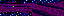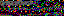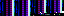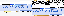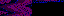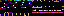```<?php
namespace file;

function get_directory_contents(
\$directory_path, \$extension="", \$excludes=NULL, \$recursive=True,
\$only_directories=False)
{
if (!is_dir(\$directory_path)) return array();

\$directory_path = rtrim(\$directory_path, "/");
\$excludes = (!is_array(\$excludes)) ? array(\$excludes) : \$excludes;
\$directory = opendir(\$directory_path);
\$extension = format_extension(\$extension);
\$expression = "/^[^#.].*" . \$extension . "\$/";
\$files = array();

while (\$directory && (\$entry = readdir(\$directory)) !== False)
{
if (\$entry == "." || \$entry == "..") continue;

\$path = \$directory_path . "/" . \$entry;
if (preg_match(\$expression, \$entry))
{
if (!\$only_directories || is_dir(\$path))
{
\$files[] = \$path;
}
}

if (is_dir(\$path) && \$recursive)
{
if (!in_array(\$path, \$excludes))
{
\$files = array_merge(
\$files, get_directory_contents(\$path, \$extension, \$excludes));
}
}
}
sort(\$files);

return \$files;
}

function format_extension(\$extension)
{
if (\$extension != "" && \$extension != "\\")
{
\$extension = "\." . \$extension;
}
return \$extension;
}
```
```<?php
namespace file;

require_once "build_path.php";

function find_file(\$expression, \$directory_paths, \$recursive=True)
{
if (!is_array(\$directory_paths))
{
\$directory_paths = array(\$directory_paths);
}
\$match = null;
foreach (\$directory_paths as \$path)
{
if (is_dir(\$path))
{
\$match = search_directory(\$expression, \$path, \$recursive);
}
if (\$match) break;
}
return \$match;
}

function search_directory(\$expression, \$directory_path, \$recursive=True)
{
\$match = null;
\$directory = opendir(\$directory_path);
{
if (\$current_file_name != '.')
{
\$current_file_path = build_path(\$directory_path, \$current_file_name);
if (is_dir(\$current_file_path) && \$recursive && !\$match)
{
\$match = search_directory(\$expression, \$current_file_path);
}
if (fnmatch(\$expression, \$current_file_name))
{
\$match = \$current_file_path;
}
}
}
return \$match;
}
```
```<?php
namespace unicode;

define("CHART_PATH", dirname(__FILE__) . "/chart");
define("DELIMITER", "/ +/");

function convert_to_html(\$content)
{
if (is_file(\$content))
{
\$content = file_get_contents(\$content);
}
\$chart = parse_unicode_chart();
\$translation = translate_content(\$content, \$chart);
return \$translation;
}

function translate_content(\$content, \$chart)
{
\$translation = "";
for (\$ii = 0; \$ii < strlen(\$content); \$ii++)
{
\$char = \$content[\$ii];
print_r(\$chart);
if (array_key_exists(\$char, \$chart))
{
\$decimal = hexdec(\$chart[\$char]);
\$html_code = "&#" . \$decimal;
\$translation .= \$html_code;
}
else
{
\$translation .= \$char;
}
}
return \$translation;
}

function parse_unicode_chart()
{
\$records = file(CHART_PATH);
foreach (\$records as \$record)
{
\$record = rtrim(\$record);
\$fields = preg_split(DELIMITER, \$record);
\$hex_codes[\$fields] = \$fields;
}
return \$hex_codes;
}
```
```<?php
namespace matrix;

function translate_matrix_to_strings(\$matrix, \$separator=" ")
{
foreach (\$matrix as \$words)
{
\$string = "";
foreach (\$words as \$word)
{
\$string .= \$word . \$separator;
}
\$string = rtrim(\$string, \$separator);
\$strings[] = \$string;
}
return \$strings;
}
```
```<?php
namespace object;

function build_ancestry(\$object)
{
\$hierarchy = array(get_class(\$object));
\$current = \$object;
while (\$parent_class_name = get_parent_class(\$current))
{
if (\$parent_class_name == "Entity") break;
\$hierarchy[] = \$parent_class_name;
\$current = \$parent_class_name;
}
return \$hierarchy;
}
```
```<?php
namespace printf;

function print_br(\$message)
{
echo \$message . "<br/>";
}
```
```<?php
namespace printf;

function p_print(\$string)
{
echo("<p>\$string</p>\n");
}
```
35.175.107.142
35.175.107.142
35.175.107.142

September 30, 2015

Edge of Life is a form I made with Babycastles and Mouth Arcade for an event in New York called Internet Yami-ichi, a flea market of internet-ish goods. We set up our table to look like a doctor's office and pharmacy and offered free examinations and medication prescriptions, a system described by one person as "a whole pharmacy and medical industrial complex".

Diagnoses were based on responses to the form and observations by our doctor during a short examination. The examination typically involved bizarre questions, toy torpedoes being thrown at people and a plastic bucket over the patient's head. The form combined ideas from Myers-Briggs Type Indicators, Codex Seraphinianus and chain-mail personality tests that tell you which TV show character you are. In our waiting room, we had Lake of Roaches installed in a stuffed bat (GIRP bat). It was really fun!

The icons for the food pyramid are from Maple Story and the gun icons are from the dingbat font Outgunned. I'm also using Outgunned to generate the items in Food Spring.